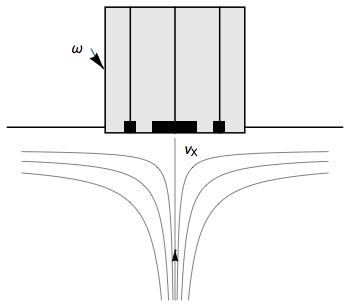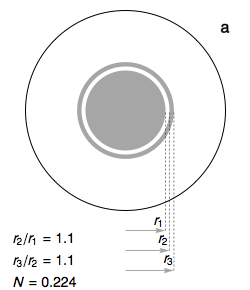Topic 5 min read# Rotating Ring Disk Electrode: an Introduction

Latest updated: June 2, 2023

Introduction

The idea for the Rotating Ring Disk Electrode (RRDE) followed the development of the Rotating Disk Electrode (RDE) and was first proposed by Frumkin in 1958 . The RRDE consists of adding a concentric ring around the RDE to collect species produced at the disk and transported away from it, following electrolyte transport lines.Figure 1: Fluid movement at a uniformly rotating ring-disk electrode. $v_\mathrm{x}$ is the axial velocity of the fluid.

The first reaction to be studied was the Oxygen Reduction Reaction (ORR). The oxygen produced at the disk through a reduction reaction was then reoxidized at the ring.

The half-reaction at the disk can be written:

$$\mathrm{A}\to\mathrm{B}+z_\mathrm{D}\mathrm{e}\tag{1}$$

Where $z_\mathrm{D}$ the number of electrons involved in the reaction at the disk, is negative in the case of a reduction and positive in the case of oxidation.

The half-reaction at the ring can be written:

$$\mathrm{B}\to\mathrm{C}+z_\mathrm{R}\mathrm{e}\tag{2}$$

Where $z_\mathrm{R}$ the number of electrons involved in the reaction at the ring, is negative in the case of a reduction and positive in the case of an oxidation.

In most cases, the ring half-reaction is the reverse disk half-reaction, but not always -this is why we need to separate the number of electrons involved in the disk and the ring reactions.

The maximum theoretical collection efficiency $N_\mathrm{max}$

When we perform Cyclic Voltammetry on the disk with the ring polarized so that the species B is being consumed, the ring current also shows a mass-transport limited current, which is smaller than the mass-transport limited current on the disk because some of the B species is being carried away from the ring by mass-transport.

The maximum theoretical collection efficiency $N_\mathrm{max}$ represents the theoretical fraction of species from the disk that is supposed to arrive on the ring. This number depends only on hydrodynamics and on the geometry of the ring-disk electrode. In Fig. 2, we can see how the ratii $r_\mathrm2/r_\mathrm1$ and $r_\mathrm3/r_\mathrm2$ can affect the value of $N_\mathrm{max}$.Figure 2: Evolution of the theoretical collection efficiency $N=N_\mathrm{max}$ as a function of the geometrical parameters of the ring-disk electrode.

From Fig. 2a to Fig. 2e, the ratio $r_\mathrm1/r_\mathrm2$ changes from 1.1 to 1.5. In each Figure 2a, 2b, 2c, 2d and 2e, the ratio $r_\mathrm3/r_\mathrm2$ changes from 1.1 to 1.5.

The expression used to calculate $N_\mathrm{max}$ is taken from the Eqs. (6.1) and (6.2) from the seminal paper by Albery and Bruckenstein .

$N_\mathrm{max}$ can be used to calculate the theoretical mass-transport-limited ring current $I_\mathrm{dR}$ from the mass-transport-limited disk current $I_\mathrm{dD}$:

$$I_\mathrm{dD}= I_\mathrm{dR}(z_\mathrm{R}/ z_\mathrm{D})N_\mathrm{max}\tag{3}\label{eq3}$$

A discrepancy from the theory can arise if the intermediate species $\mathrm{B}$ is unstable and is chemically transformed into $\mathrm{Z}$ following:

$$\mathrm{B}\overset{k}\to\mathrm{Z}\tag{4}\label{eq4}$$

Where $k$ is the homogeneous rate constant. Studying the discrepancy from Eq.$\eqref{eq3}$ at various rotation rates provides a way of measuring $k$ as described in the series of papers by Albery and Bruckenstein [3-5]. RRDE experiments require the independent control of two potentiostat channels that manage two working electrodes sharing the same reference and counter electrodes. For more details on the connections needed please see the article on Multi-electrode measurement.

Finally, an interactive Mathematica Demonstration is available representing $N_\mathrm{max}$ as a function of the ring-disk electrode geometry and the adimensional $I_\mathrm{dD}, I_\mathrm{dR} \,vs. E_\mathrm{D}$ curves .

Bi-pot measurements

RRDE experiments require to independently control two potentiostat channels that manage two working electrodes sharing the same reference and counter electrodes. For more details on the connections needed please see the topic on Multi-electrode measurement.

Bi-pot techniques are readily available in EC-Lab® to perform RRDE measurements. Please see more details in Application Note 7.

 A. Frumkin, L. Nekrasov, B. Levich, JU. Ivanov, J. Electroanal. Chem., 1 (1959) 84.

 W.J. Albery and S. Bruckenstein, Trans. Faraday Soc., 62 (1966) 1920.

 W. J. Albery and S. Bruckenstein, Trans. Faraday Soc., 62 (1966) 1946.

 W. J. Albery and S. Bruckenstein, Trans. Faraday Soc., 62 (1966) 2584.

 W. J. Albery and S. Bruckenstein, Trans. Faraday Soc., 62 (1966) 2596.

## Related products

Work smarter. Not harder.

Tech-tips, theory, latest functionality, new products & more.

No thanks!